Publicité

# Mathematical support for preventive maintenance periodicity optimization of radiocommunication facilities

18 Feb 2016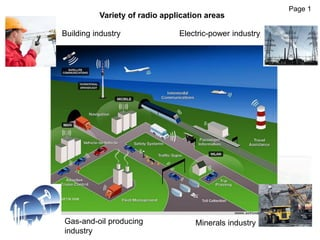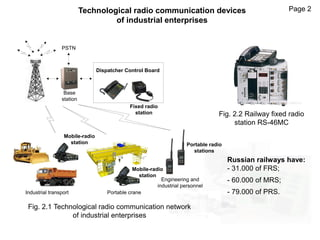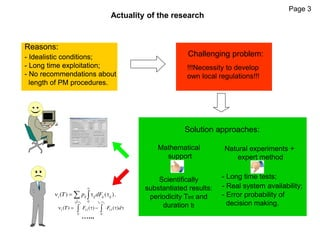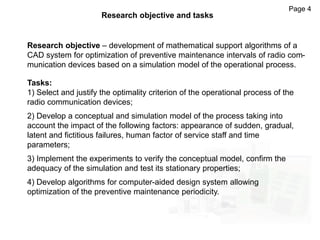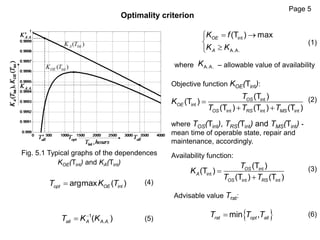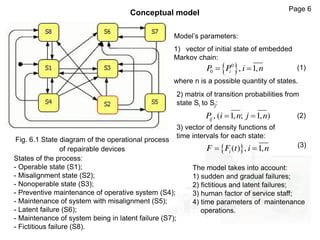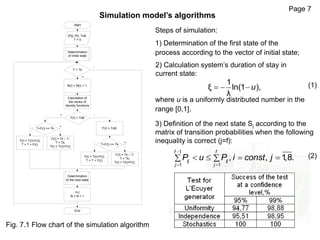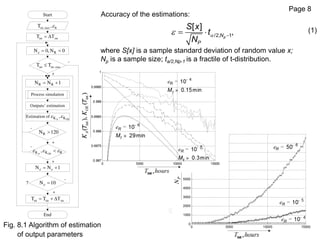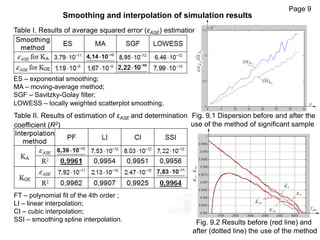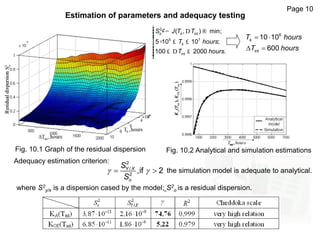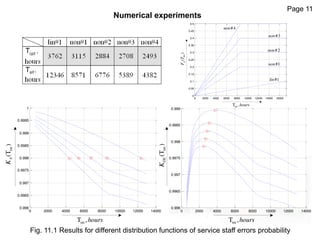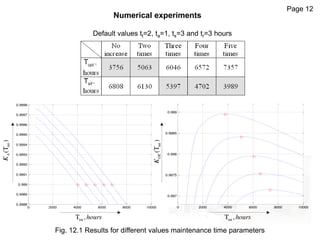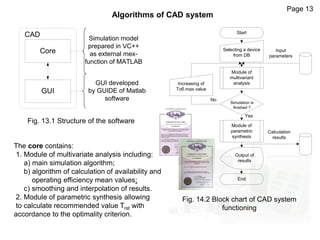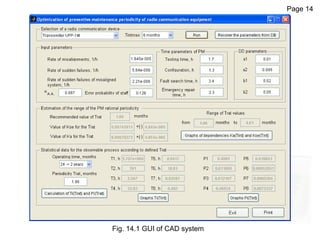1 sur 15
Publicité

### Mathematical support for preventive maintenance periodicity optimization of radiocommunication facilities

1. Mathematical support for preventive maintenance periodicity optimization of radio communication facilities The Ministry of Transport of the Russian Federation The Federal Agency of Railway Transport Omsk State Transport University (OSTU) Granada, 2016 Alexander Lyubchenko – Associate Prof. of the Dept. Information and communication systems and data security
2. Variety of radio application areas Page 1 Building industry Gas-and-oil producing industry Electric-power industry Minerals industry
3. Page 2Technological radio communication devices of industrial enterprises Fig. 2.1 Technological radio communication network of industrial enterprises Base station PSTN Dispatcher Control Board Industrial transport Portable crane Engineering and industrial personnel Mobile-radio station Mobile-radio station Portable radio stations Fixed radio station Fig. 2.2 Railway fixed radio station RS-46MC Russian railways have: - 31.000 of FRS; - 60.000 of MRS; - 79.000 of PRS.
4. Page 3 Actuality of the research Reasons: - Idealistic conditions; - Long time exploitation; - No recommendations about length of PM procedures. Challenging problem: Solution approaches: Natural experiments + expert method - Long time tests; - Real system availability; - Error probability of decision making. Mathematical support Scientifically substantiated results: periodicity Tint and duration tt Reasons: - Idealistic conditions; - Long time exploitation; - No recommendations about length of PM procedures. !!!Necessity to develop own local regulations!!! 0 ( ) ( ).i ij ij ij ij j T p dF       о д о д 1 12 13 0 0 ( ) ( ) ( ) t t t t T F F d           …...
5. Research objective – development of mathematical support algorithms of a CAD system for optimization of preventive maintenance intervals of radio com- munication devices based on a simulation model of the operational process. Page 4 Research objective and tasks Tasks: 1) Select and justify the optimality criterion of the operational process of the radio communication devices; 2) Develop a conceptual and simulation model of the process taking into account the impact of the following factors: appearance of sudden, gradual, latent and fictitious failures, human factor of service staff and time parameters; 3) Implement the experiments to verify the conceptual model, confirm the adequacy of the simulation and test its stationary properties; 4) Develop algorithms for computer-aided design system allowing optimization of the preventive maintenance periodicity.
6. Optimality criterion Page 5 Fig. 5.1 Typical graphs of the dependences KOE(Tint) and KA(Tint) where – allowable value of availability Objective function KOE(Tint): where TOS(Tint), TRS(Tint) and TMS(Tint) - mean time of operable state, repair and maintenance, accordingly. Availability function: Advisable value Trat: (1) (3) (4) (5) (6)     int A.A. (T ) maxOE A K f K K A.A.K int int int int int (T ) (T ) (T ) (T ) (T ) OS OE OS RS MS T K T T T    (2) int int int int (T ) (T ) (T ) (T ) OS A OS RS T K T T   intargmax ( )opt OET K T 1 . .( )all A A AT K K   min ,rat opt allT T T
7. Fig. 6.1 State diagram of the operational process of repairable devices Conceptual model Model’s parameters: 1) vector of initial state of embedded Markov chain:  0 0 , 1,iP P i n  where n is a possible quantity of states. 2) matrix of transition probabilities from state Si to Sj: , ( 1, ; 1, )ijP i n j n  3) vector of density functions of time intervals for each state:  ( ) , 1,iF F t i n  States of the process: - Operable state (S1); - Misalignment state (S2); - Nonoperable state (S3); - Preventive maintenance of operative system (S4); - Maintenance of system with misalignment (S5); - Latent failure (S6); - Maintenance of system being in latent failure (S7); - Fictitious failure (S8). (1) (2) (3) Page 6 The model takes into account: 1) sudden and gradual failures; 2) fictitious and latent failures; 3) human factor of service staff; 4) time parameters of maintenance operations.
8. Page 7 Simulation model’s algorithms Start T < Tk |Pij|, P0, Tоб, Т = 0 - + N(I) = N(I) + 1; Determination of initial state Calculation of the vector of density functions F(I) < Tоб -+ T+F(I) >= Tk - + F(I) = Tk – T; T = Tk; T(I) = T(I)+F(I); T(I) = T(I)+F(I); T = T + F(I); F(I) = Tоб; T+F(I) >= Tk - + F(I) = Tk – T; T = Tk; T(I) = T(I)+F(I); T(I) = T(I)+F(I); T = T + F(I); Determination of the next state I=J N = N + 1 End Fig. 7.1 Flow chart of the simulation algorithm Steps of simulation: 1) Determination of the first state of the process according to the vector of initial state; 2) Calculation system’s duration of stay in current state: 1 ξ ln(1 ), λ u   where u is a uniformly distributed number in the range [0,1]. 3) Definition of the next state Sj according to the matrix of transition probabilities when the following inequality is correct (j=f): 1 1 1 , , 1,8. f f ij ij j j P u P i const j         (1) (2)
9. Page 8 Fig. 8.1 Algorithm of estimation of output parameters - + Process simulation Outputs’ estimation + + - - - + End Start 7 int intT Т  int.max RT , RN 0,N 0   int int.maxT T R RN N 1  A OEK K, Estimation of RN 120 A OEK K R,   N N 1   N 10  int int intT T Т   Accuracy of the estimations: /2, 1 [ ] ,pN P S x t N    (1) where S[x] is a sample standard deviation of random value x; Np is a sample size; ta/2,Np-1 is a fractile of t-distribution.
10. Page 9 Smoothing and interpolation of simulation results ES – exponential smoothing; MA – moving-average method; SGF – Savitzky-Golay filter; LOWESS – locally weighted scatterplot smoothing. FT – polynomial fit of the 4th order ; LI – linear interpolation; CI – cubic interpolation; SSI – smoothing spline interpolation. Table I. Results of average squared error (εASE) estimation Table II. Results of estimation of εASE and determination coefficient (R2) Fig. 9.1 Dispersion before and after the use of the method of significant sample Fig. 9.2 Results before (red line) and after (dotted line) the use of the method
11. Page 10 Estimation of parameters and adequacy testing 2 int 5 7 int ( , ) min; 5 10 10 ; 100 2000 . o k k S J T T T hours T hours ìï ¢= D ®ïïïï × £ £í ïïï £ D £ïïî     6 int 10 10 600 kT hours T hours Fig. 10.1 Graph of the residual dispersion Fig. 10.2 Analytical and simulation estimations Adequacy estimation criterion:    2 / 2 ,if 2Y X o S S where S2 y/x is a dispersion cased by the model; S2 o is a residual dispersion. the simulation model is adequate to analytical.
12. Page 11 Numerical experiments 0 2000 4000 6000 8000 10000 12000 14000 0.996 0.9965 0.997 0.9975 0.998 0.9985 0.999 0 2000 4000 6000 8000 10000 12000 14000 0.996 0.9965 0.997 0.9975 0.998 0.9985 0.999 0.9995 1 intT ,hours intT ,hours int(T)OEK int(T)AK 0 2000 4000 6000 8000 10000 12000 14000 16000 0 0.05 0.1 0.15 0.2 0.25 0.3 0.35 0.4 0.45 0.5 intT ,hours int()pFT #1lin #1non #2non #3non #4non Fig. 11.1 Results for different distribution functions of service staff errors probability
13. Page 12 Numerical experiments Fig. 12.1 Results for different values maintenance time parameters 0 2000 4000 6000 8000 10000 0.997 0.9975 0.998 0.9985 0.999 0 2000 4000 6000 8000 10000 0.9988 0.9989 0.999 0.9991 0.9992 0.9993 0.9994 0.9995 0.9996 0.9997 0.9998 intT ,hours intT ,hours int(T)OEK int(T)AK Default values tt=2, ta=1, ts=3 and tr=3 hours
14. Module of multivariant analysis No Yes Increasing of Тоб.max value Start Selecting a device from DB Simulation is finished ? Module of parametric synthesis Calculation results Output of results End Input parameters Algorithms of CAD system Fig. 14.2 Block chart of CAD system functioning Core GUI CAD Simulation model prepared in VC++ as external mex- function of MATLAB GUI developed by GUIDE of Matlab software Page 13 Fig. 13.1 Structure of the software The core contains: 1. Module of multivariate analysis including: a) main simulation algorithm; b) algorithm of calculation of availability and operating efficiency mean values; c) smoothing and interpolation of results. 2. Module of parametric synthesis allowing to calculate recommended value Trat with accordance to the optimality criterion.
15. Fig. 14.1 GUI of CAD system Page 14
Publicité